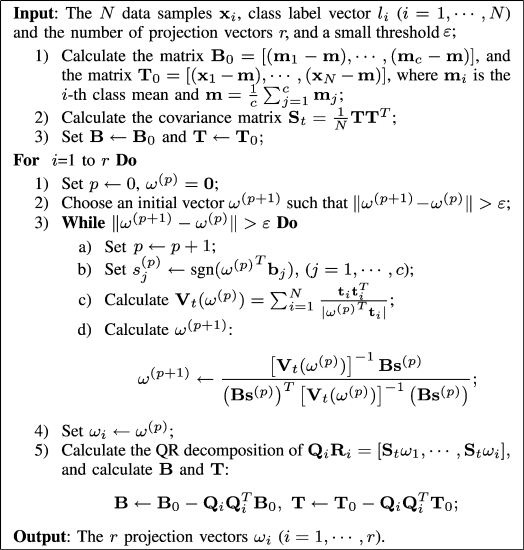# L1-Norm Kernel Discriminant Analysis Via Bayes Error Bound Optimization for Robust Feature Extraction### Abstract

A novel discriminant analysis criterion is derived in this paper under the theoretical framework of Bayes optimality. In contrast to the conventional Fisher’s discriminant criterion, the major novelty of the proposed one is the use of L1 norm rather than L2 norm, which makes it less sensitive to the outliers. With the L1-norm discriminant criterion, we propose a new linear discriminant analysis (L1-LDA) method for linear feature extraction problem. To solve the L1-LDA optimization problem, we propose an efficient iterative algorithm, in which a novel surrogate convex function is introduced such that the optimization problem in each iteration is to simply solve a convex programming problem and a close-form solution is guaranteed to this problem. Moreover, we also generalize the L1-LDA method to deal with the nonlinear robust feature extraction problems via the use of kernel trick, and hereafter proposed the L1-norm kernel discriminant analysis (L1-KDA) method. Extensive experiments on simulated and real data sets are conducted to evaluate the effectiveness of the proposed method in comparing with the state-of-the-art methods.

Type
Publication
IEEE Transactions on Neural Networks and Learning Systems